Home News What is baudline? Screenshots Download FAQ Manual Search Solutions Mystery Signal Contact
measurements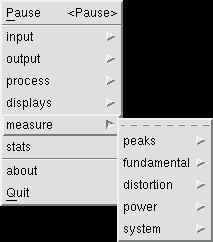The measurement windows automatically display a wide variety of signal parameters, internal baudline variables, and calculated statistics.  Their goal is to quickly and accurately report numerical values of things you might want to measure in baudline.  In fact the values they report are far more accurate than can be measured manually with the mouse.  The units range from Hz, dB, PSD, percent, semitones, RPM, counts, and time.  There are four basic families of measurement windows and they each have their own unique set of features, constraints, and behaviors.  They are: The window below is peak frequency measurement window of a 2-channel stereo signal.  Note that baudline can handle up to nine channels of signals.The design philosophy of the measurement windows is for the user to select a layout -session, configure the baudline mission settings, choose a couple measurement windows, position them horizontally or vertically, perform some signal analysis and then exit.  This way many different complex and sophisticated test and measurement scenarios can be saved for later use. The measurement windows use a shared menu concept.  That means options selected in one window apply to all the measurement windows.  Changing the source, precision, font size, or any of the other options will have an immediate effect on other windows.  This was done to simplify configuration and make the implementation manageable.  Below is a typical measurement window's popup menu:Paste Select and paste a section of sample data into the measurement windows for a long duration summation while in the Pause mode.  This reduces the variance (error) and results in a more accurate measurement.  The paste operation here is very much like the paste feature in the Average window.  AC coupling The AC coupling option removes the DC component from all spectral based measurements.  This is useful when a strong 0 Hz frequency value is causing improper peak detection or contaminating any distortion measurements such as THD or ENOB.source Select the spectrum or the average window as the measurement source.  The Spectrum source is dynamic in that it tracks what is being recorded, played, or pointed to in the pause mode.  The Average source tracks the current Average bank (F#).  Clear the Average bank or select a different one and the measurement changes.  Each source has its benefits and drawbacks.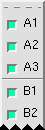channel This option selects which channels should be enabled for viewing when the source is the spectrum mode.  The default setting is to view all channels.  The intended use of this menu is to reduce the number of displayed measurement channels for aesthetic or duplicate reasons.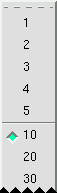record averaging This option is only valid when the source is set to Spectrum and baudline is in the Record mode.  The selected value represents the number of slices that should be averaged.  The number of record averaging slices can range from 1 to 5000.  The amount of time this equals is a function of sample rate and scroll control overlap setting.  A smaller number results in a faster changes and a more immediate response which is useful for non stationary signals.  A larger setting means a longer average which has the effect of stabilizing the update jitter (digit spinning).  This can make the display more readable and also improve the accuracy (precision) of the measurement. This averaging option can be thought of as reducing the measurement variance.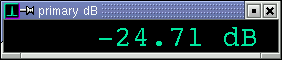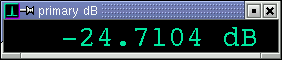precision Use the left and right arrow keys to change the number of precision decimal places.  This control has no effect on internal accuracy.  It simply sets the rounding and truncation decimal position.  Many factors contribute to measurement error such as noise, signal strength, signal jitter, and floating point numeric effects.  Depending on the situation and signal source, the number of significant decimal digits can vary from zero to more than six.  A good general rule to follow while recording is that "spinning digits are not valid digits." There are some operational methods that can be utilized by the user to increase measurement precision.  For example:  Reducing the spectral variance by averaging is a good thing for noisy or weak signals.  This is accomplished either with the Drift Integrator or the Average window.  For strong signals, the Spectrum window source might produce better results than the Average window source due to numeric accumulation noise.  The record averaging option mentioned above works good for What works good for one type of measurement might not be the best approach for a different type of measurement.  Using calibrated test signals (external or internal Tone Generator loopback) are the best method to use for determining significant digits. Note that reducing the spectral variance is different than reducing the variance of the measurement (see record averaging option).  In the Drift Integrating while recording case, both forms of averaging can be done at the same time.  This isn't inherently good or bad, it is just an interesting combination that the user should be aware of.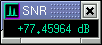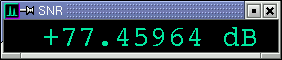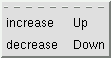font size Use the up and down arrow keys or the mouse scroll wheel to increase or decrease the font size.  The size of the measurement windows can be made full width or larger for easy viewing from across the room.

peaksThe peaks family consists of primary, secondary, and delta measurements.  Units can be Hz, dB, and PSD.  The peak measurements have all of the popup menu options listed above (source, channel, record averaging, precision, and font size).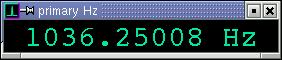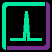primary (Hz dB PSD) This is the strongest spectral peak.  The dB and the PSD measurements match the ruler and on-screen-display values which means that they are calculated directly from the numeric values of the largest transform bin.  The Hz measurement also uses the largest transform bin but it employs the uHz-rotator algorithm that operates in the complex domain to calculate an extremely accurate estimate of the frequency.  Under good conditions (strong signal and low noise) the frequency accuracy can exceed 8 significant digits (~.000001 Hz).  All of the measurement windows that work with Hz utilize the uHz-rotator algorithm.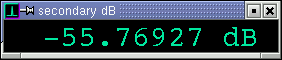secondary (Hz dB PSD) This is the second strongest spectral peak.  It can be either to the left or the right of the primary peak.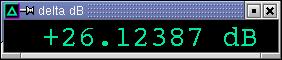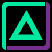delta (Hz dB PSD) This is the difference between the primary and the secondary peaks.  The delta dB measurement works the same as if you manually used the delta measurement bars.  The delta Hz measurement is similar but it uses the uHz-rotator algorithm for an extremely accurate delta Hz measurement.

fundamental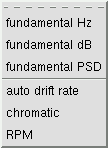The fundamental family of measurements utilizes a concept of the fundamental and its harmonics.  The fundamental is the first harmonic and the second, third, fourth, ... harmonics are integer multiples of the fundamental frequency.  See the harmonic helper bars for more educational information on harmonics.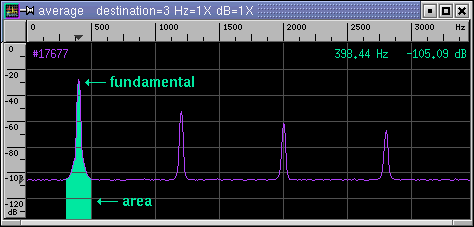fundamental rule The fundamental measurements have the same popup menu options that the peaks measurements have plus this fundamental rule menu option.  The default option is to use the primary Hz as the fundamental, with this rule the strongest peak is treated as the fundamental.  The max in Hz range option calculates the strongest peak that is within a bounded frequency range and uses that as the fundamental.  The multiple mouse options track the frequency value that the mouse in the Spectrum or Average window is pointing to.  The exact option uses the bin number exactly and is the crudest setting.  The float option is like the exact setting except that it uses the uHz-rotator algorithm for a more accurate frequency value.  The climb option is like the float option except that it also climbs the highest adjacent peak.  The climb algorithm is the most useful of the mousing options, just point near the peak and an accurate answer is the result.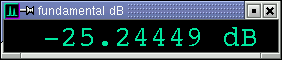fundamental (Hz dB PSD) Similar to the peaks measurements but different in two significant ways.  The first difference is tracking, the fundamental Hz measurement is the same as the primary Hz measurement when the fundamental rule is set to "use primary Hz" but it becomes something else entirely when a different rule is used.  The second difference is that the dB and PSD measurements calculate the area under the curve as shown in the average picture above.  A major benefit of the area summation is that it solves the window leakage problem.  Peak energy is now constant and no longer related to frequency and window function. For an educational experiment use the Tone Generator with loopback and step or sweep the frequency.  The primary dB measurement will fluctuation as a function of frequency.  The fundamental dB measurement will remain constant for all frequency values.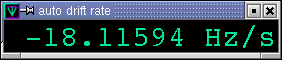auto drift rate Reports the auto drift rate in Hz per second.  Probe the drift rate of specific bins by changing the fundamental rule to mouse exact.  Useful for measuring Doppler drift rate.  The Drift Integrator's auto drift feature needs to be enabled for this measurement to work.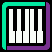chromatic Display the fundamental frequency in units of the chromatic scale.  Useful for transcription and instrument tuning.  The display format is octave number, semitone note, and cents.  There are 100 cents per semitone and the notes of the chromatic scale are { A Bb B C C# D Eb E F F# G G# }.   Semitones get rounded up or down at the 50 cent value.  This measurement window can be used in conjunction with the piano keys controller.calibration The chromatic measurement window's A2 calibration can set to one of the several fixed frequency values.  Or it can be calibrated to any arbitrary Hz value with the grab tone generator option which takes the current main frequency value in the tone generator window and sets A2 to that.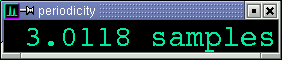periodicity Display the fundamental frequency in the units samples of periodicity.  A value of 3 means that some signal characteristic repeats every 3 samples.  This measurement window is useful for situations where the sample rate value isn't appropriate such as with nucleotide or raw binary data streams.  Formula: ```period = sample rate / Hz ```RPM Revolutions per minute.  Like a tachometer.  Designed to calculate the rotational rate of many different types of spinning machinery.  Proper configuration of machine parameters must be set for accurate RPM measurements.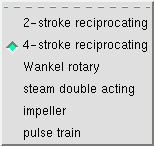cycle Describe the cycle type of the machine under test.  2-stroke reciprocating - internal combustion engine; lawn mowers, leaf blowers, weed wackers, chainsaws, dirt bikes 4-stroke reciprocating - internal combustion engine; automobiles, motorcycles Wankel rotary - internal combustion engine; automobiles steam double acting - steam driven train engine impeller - propellers, fans, pumps pulse train - single pulse; crankshaft sensor, capacitive discharge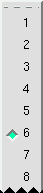cylinders The cycle factor.  Range from 1 to 24.  Units are a function of cycle type and can be cylinders, rotors, blades, or pulses.

distortion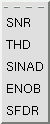Noise and distortion measurements.  A subset of the fundamental family of measurements.  Utilizes the fundamental rule menu option.  The harmonics and noise floor are calculated from the fundamental frequency, the ratios between these make up the distortion family of measurements.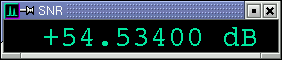SNR Signal to Noise Ratio where the noise excludes the harmonics.  Units are decibels (dB).  Larger SNR equals cleaner signal.  When the SNR is zero dB the amount of signal equals the amount of noise.  Negative SNR can be thought of as how many dB the signal is beneath the noise floor.  There are two interesting limits on the fundamental frequency range: When the fundamental is extremely low in frequency all frequencies greater than the fundamental are considered harmonics.  In this case there is no noise (zero) and the SNR display is +infinity.  When the fundamental is greater than half the Nyquist frequency the harmonics are zero and the distortion measurements of SNR and SINAD are equal. Formula: ```SNR = RMS fundamental / RMS (noise excluding harmonics) ```THD Total Harmonic Distortion is a measure of nonlinearity.  Noise and nonlinearity are different things.  THD is the ratio of harmonic energy to fundamental energy.  The units are decibels (dB) and percent (%).  A THD of 0 dB is the same as 100% THD.  Smaller THD (more negative) equals a cleaner signal.  Caveats: When the fundamental is greater than half the Nyquist frequency the harmonics are zero and the THD display is -infinity. Like with SNR, when the fundamental is extremely low in frequency there is no noise (zero).  This means everything that is not the fundamental is considered harmonics.  When this happens the value of THD is the same as the SINAD measurement but negative. The THD percent measurement is a power ratio where a THD of -20 dB is 1%.  This should not be confused with an amplitude ratio where a THD of -40 dB is 1%.  Both are valid ways of expressing THD as a percent.  Baudline uses the power ratio method.  Use the THD dB value if you wish to reduce any chance of ambiguous interpretation. Formula: ```THD = RMS harmonics / RMS fundamental ```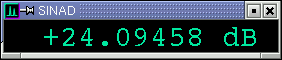SINAD Signal to Noise and Distortion Ratio where the noise includes the harmonics.  Similar to SNR but the harmonics are treated as part of the noise.  Negative SINAD represents dB below the noise floor.  Interesting note: When the fundamental is greater than half the Nyquist frequency the harmonics are zero and the distortion measurements of SNR and SINAD are equal. Formula: ```SINAD = RMS fundamental / RMS (noise including harmonics) = RMS fundamental / RMS (noise + harmonics) = 1. / RMS (harmonics / fundamental + noise / fundamental) = -RMS (THD - SNR) where (-) signifies inversion (/) ``` Leads to these two interesting relationships in dB space: ```SINAD = -20. * log10(sqrt(10^(THD/10.) + 10^(-SNR/10.))) THD+N = -SINAD ```ENOB Effective Number of Bits.  Units are bits (see power of two).  Calculated from SINAD.  Can be used to describe the quality of an ADC (analog to digital converter).  Noise such as dither reduces the effective bits of a system.  The meaning of negative bits is unknown. Formula: ```ENOB = (SINAD - 20. * log10(sqrt(1.5))) / (20. * log10(2.)) = (SINAD - 1.7609 dB) / 6.0206 dB ```SFDR Spurious Free Dynamic Range.  A ratio of the signal to the strongest non signal element (spurious peak).  Units are dB.  Unlike SNR and THD, harmonic relationships are not required and the second largest peak can be either to the left or right of the fundamental. Formula: ```SFDR = RMS fundamental / RMS second largest peak ``` For a further in depth analysis of this topic see the sine distortion app note.

power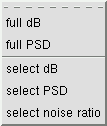The power family of measurements operate on a bounded contiguous region of spectral bins.  Either all of the spectral bins for the full case, a user specified range for the select case, or the relationship between the select and the full measurements for the ratio case.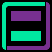full (dB PSD) This measurement is the area summation of all the spectral bins.  It is a total power measurement.  Units are dB or volts^2 for the PSD version. Formula: ```full = RMS (fundamental + harmonics + noise) ```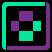select (dB PSD) This measurement is the area summation of a selected frequency range of spectral bins.  It is a total power measurement.  Units are dB or volts^2 for the PSD version.  This measurement will always be equal to or less than the full power measurement. Formula: ```select = RMS (selected frequency range) ```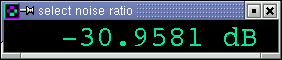select noise ratio Select to Full power ratio.  Like SNR or SINAD except that the select (fundamental) is now user definable.  Units are in dB.  The region that is within the select zone is considered signal while the region not in the select zone is considered noise hence the term select noise ratio. Formula: ```ratio = RMS select / RMS (full - select) = RMS signal / RMS noise ```

systemThe system family of measurement windows consist of time domain counts like clips, user selected ranges, and clock time.  It is a catch all category for reporting internal baudline parameters, user settings, and other measurements that don't fit into the above defined measurement classes.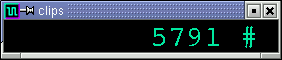clips Also known as over saturation, sample clipping is a cause of harmonic distortion.  The clips measurement window counts the number of samples that have the value of -32768 or +32767.  The more severe the clipping the higher or faster the count.  The Histogram and the Waveform window can be used to verify or locate the time position of the clipping.  This is a handy window to keep open while recording a live signal source because it provides fast and accurate statistics. A section of samples can be selected and pasted into the clips window.  The #count can also be manually cleared from the popup menu.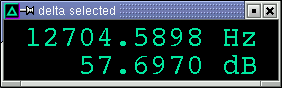delta selected This window displays the delta selected measurement from either the average, spectrogram, spectrum, or waveform windows.  For spectral measurements the delta units are Hz and dB.  For time measurements the delta units are seconds and (1/seconds) Hz which is useful for periodicity measurements.  A measurement is held on the delta display until another measurement is done.  The measurement can now easily be written down or used as an on screen reminder.  Also if the numeric precision digits is set at a high value then the delta selected measurement window will display more significant numeric digits than the on-screen-displays can report.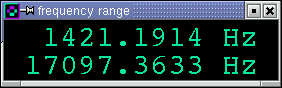frequency range This window displays the last selected frequency range from either the average or the spectrum windows.  The units are in Hz with the upper and lower values representing the start and end frequencies.  The fundamental and distortion class measurement windows use this bounded frequency range when the fundamental rule is set to the "max in Hz range" option.  The select power measurements also use this bounded frequency range as a basis for their summed power calculations.  A range measurement is held until another frequency selection is done.  Note that the delta selected measurement window calculates and displays the corresponding (end - start) Hz delta value.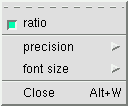ratio The ratio toggle option adds another row to the frequency range measurement window.  The new measurement displayed is the ratio of the end Hz to the start Hz value.  This is a very useful feature for determining harmonic ratios that are not exact integer values.  Pressing the / (slash) key while the mouse is in the frequency range window flips the numerator and the denominator for a 1/x effect.  The units are "/" and "\" which indicate ratio and the direction of the division. Formula: ```ratio = end Hz / start Hz = start Hz \ end Hz ```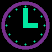cursor time The format is HH:MM:SS.uuuuuu where HH is hours, MM is minutes, SS is seconds, and .uuuuuu is microseconds.  The time reported is relative time from the 0 ms position which is at the top for loaded files and the bottom for recorded signals.  The spectrogram and the waveform selector bars are the source of time position information.  So when the mouse is moved in either window this measurement will reflect that time position.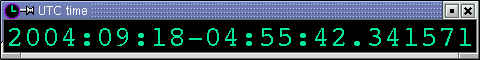UTC time This measurement reports absolute time as either clock time or elapsed time depending on the particular baudline mode of operation.  When used with the -utc command line option the absolute UTC time is tracked while in both record and pause modes.  When used without the -utc command line option; recording reports elapsed record duration, and a mode change to pause grabs the computer's UTC clock time which is used as a basis of absolute time for the last sample recorded.  The format is YYYY:MM:DD-HH:MM:SS.uuuuuu where YYYY is years, MM is months, DD is days, HH is hours, MM is minutes, SS is seconds, and .uuuuuu is microseconds.  Pause mode operates like the cursor time measurement in that the spectrogram and the waveform selector bars are the source of time position information.  Note that the microseconds value is accurate to the sample interval.